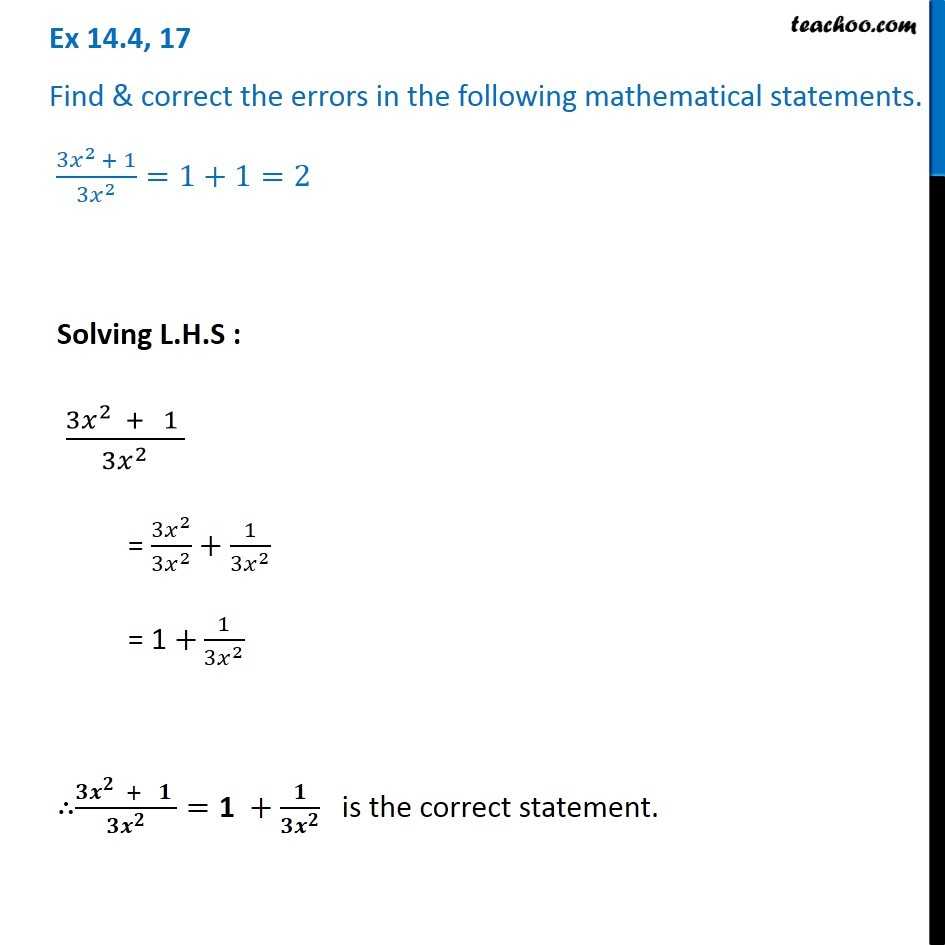Ex 14.4

Chapter 14 Class 8 Factorisation
Serial order wiseGet live Maths 1-on-1 Classs - Class 6 to 12

### Transcript

Ex 14.4, 17 Find & correct the errors in the following mathematical statements. (〖3𝑥〗^(2 )+ 1)/〖3𝑥〗^2 =1+1=2 Solving L.H.S : (3𝑥^2 + 1 )/(3𝑥^2 ) = (3𝑥^2)/〖3𝑥〗^2 +1/(3𝑥^2 ) = 1 +1/(3𝑥^2 ) ∴(𝟑𝒙^𝟐 + 𝟏 )/(𝟑𝒙^𝟐 )="1 "+𝟏/(𝟑𝒙^𝟐 ) is the correct statement.